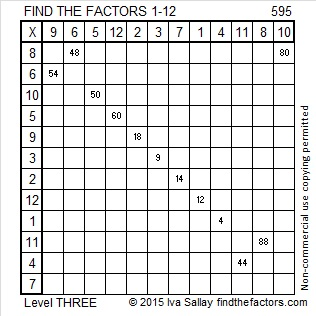# 1638 Factors and Multiples

### Mathematical Musings:

I like the way this tweet shows familiar relationships of several unfamiliar math terms.

Recalling that MANY people confuse factors with multiples, I was inspired to make something similar that will hopefully help people to know which is which: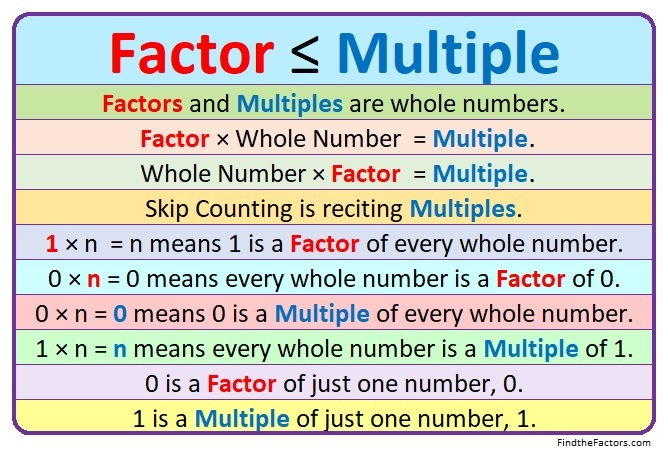### Factors of 1638:

1638 is even, so it is divisible by 2.
1
+ 8 = 9 and 6 + 3 = 9, so 1638 is divisible by 9.

• 1638 is a composite number.
• Prime factorization: 1638 = 2 × 3 × 3 × 7 × 13, which can be written 1638 = 2 × 3² × 7 × 13.
• 1638 has at least one exponent greater than 1 in its prime factorization so √1638 can be simplified. Taking the factor pair from the factor pair table below with the largest square number factor, we get √1638 = (√9)(√182) = 3√182.
• The exponents in the prime factorization are 1, 2, 1, and 1. Adding one to each exponent and multiplying we get (1 + 1)(2 + 1)(1 + 1)(1 + 1) = 2 × 3 × 2 × 2 = 24. Therefore 1638 has exactly 24 factors.
• The factors of 1638 are outlined with their factor pair partners in the graphic below.### More about the Number 1638 and Today’s Puzzle:

1638₁₀ = 666₁₆ because 6(16² + 16¹ + 16º) = 6(256 + 16 + 1) = 6(273) = 1638.

1638 is the hypotenuse of a Pythagorean triple:
630-1512-1638.Solution:
It may be a little confusing because of the words greatest and least, but remember factor ≤ multiple so
Greatest Common Factor < Least Common Multiple.
Or simply, GCF < LCM.

Since these numbers have several digits, skip counting to find common multiples isn’t practical. An easy way to solve the puzzle is to pay attention to the exponents in the prime factorizations:
630 = 2 × 3² × 5 × 7,
1512 = 2³ × 3³ × 7, and
1638 = 2 × 3² × 7 × 13.

Rewrite the prime factorizations to contain all the bases used in any of the prime factorizations with the appropriate exponents:
630 = 2¹ × 3² × 5¹ × 7¹ × 13º,
1512 = 2³ × 3³ × 5º × 7¹ × 13º, and
1638 = 2¹ × 3² × 5º × 7¹ × 13¹.

Write the bases using the SMALLEST exponents for the Greatest Common Factor:
GCF = 2¹ × 3² × 5º × 7¹ × 13º = 2 × 3² × 7 = 126.

Write the bases using the LARGEST exponents for Least Common Multiple:
LCM = 2³ × 3³ × 5¹ × 7¹ × 13¹ = 98280.
(Aren’t you glad we didn’t skip count to find it!)

Is GCF < LCM?
126 < 98280. Most certainly!

As you might expect, 630-1512-1638 is 126 times (5-12-13).

# 782 and Level 1

• 782 is a composite number.
• Prime factorization: 782 = 2 x 17 x 23
• The exponents in the prime factorization are 1, 1, and 1. Adding one to each and multiplying we get (1 + 1)(1 + 1)(1 + 1) = 2 x 2 x 2 = 8. Therefore 782 has exactly 8 factors.
• Factors of 782: 1, 2, 17, 23, 34, 46, 391, 782
• Factor pairs: 782 = 1 x 782, 2 x 391, 17 x 46, or 23 x 34
• 782 has no square factors that allow its square root to be simplified. √782 ≈ 27.96426.Here’s today’s puzzle:Print the puzzles or type the solution on this excel file: 12-factors-782-787

—————————————

OEIS.org informs us that the sum of the factors of 782 is not only a perfect square number but also a perfect 4th power.

Specifically, 1 + 2 + 17 + 23 + 34 + 46 + 391 + 782 = 1296, which happens to be 6^4.

23 is one of the factors of 782, and here are three interesting number facts about the number 782 that involve the number 23:

• 23 + 24 + 25 + 26 + 27 + 28 + 29 + 30 + 31 + 32 + 33 + 34 + 35 + 36 + 37 + 38 + 39 + 40 + 41 + 42 + 43 + 44 + 45 = 782. (That’s 23 consecutive numbers beginning with the number 23.)
• 782 is the 23rd pentagonal number because 23(3⋅23 – 1)/2 = 782.
• 782 is palindrome NN in BASE 33. (N is 23 base 10.) Note that 23(33) + 23(1) = 782

Because 17 is one of its factors, 782 is the hypotenuse of Pythagorean triple 368-690-782.

Thus, 368² + 690² = 782².

Also since 17 x 46 = 782, the greatest common factor of 368, 690 and 782 is 46.

782 is also the sum of three squares six different ways:

• 27² + 7² + 2² = 782
• 26² + 9² + 5² = 782
• 25² + 11² + 6² = 782
• 22² + 17² + 3² = 782
• 19² + 15² + 14² = 782
• 18² + 17² + 13² = 782

—————————————# 674 and Level 1

674 is the hypotenuse of the Pythagorean triple 350-576-674. What is the greatest common factor of those three numbers?

674 is a leg in exactly one Pythagorean triple: 674-113568-113570, and those three numbers have the exact same greatest common factor as the triple above.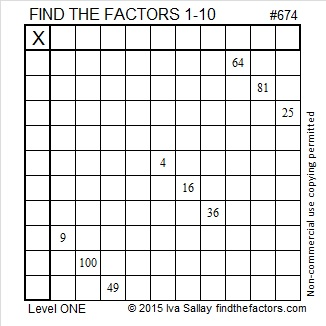Print the puzzles or type the solution on this excel file: 10 Factors 2015-11-09

—————————————————————————————————

• 674 is a composite number.
• Prime factorization: 674 = 2 x 337
• The exponents in the prime factorization are 1 and 1. Adding one to each and multiplying we get (1 + 1)(1 + 1) = 2 x 2 = 4. Therefore 674 has exactly 4 factors.
• Factors of 674: 1, 2, 337, 674
• Factor pairs: 674 = 1 x 674 or 2 x 337
• 674 has no square factors that allow its square root to be simplified. √674 ≈ 25.96151.—————————————————————————————————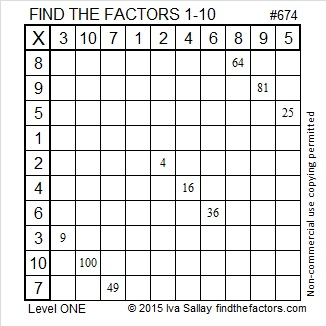# 655 and Level 6

655  is the hypotenuse of the Pythagorean triple 393-524-655. What is the greatest common factor of those three numbers?

655 is a leg in exactly three triples. One of them is primitive; the rest are not. Which is which?

• 655-1572-1703
• 655-42900-42905
• 655-214512-214513

Which of 655’s factors are the greatest common factors of each of the two that are not primitive?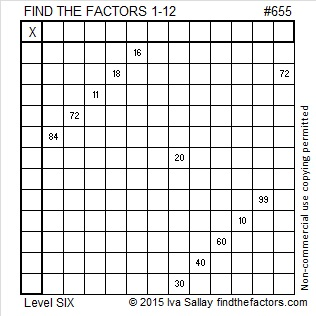Print the puzzles or type the solution on this excel file: 12 Factors 2015-10-19

—————————————————————————————————

• 655 is a composite number.
• Prime factorization: 655 = 5 x 131
• The exponents in the prime factorization are 1 and 1. Adding one to each and multiplying we get (1 + 1)(1 + 1) = 2 x 2 = 4. Therefore 655 has exactly 4 factors.
• Factors of 655: 1, 5, 131, 655
• Factor pairs: 655 = 1 x 655 or 5 x 131
• 655 has no square factors that allow its square root to be simplified. √655 ≈ 25.592968.—————————————————————————————————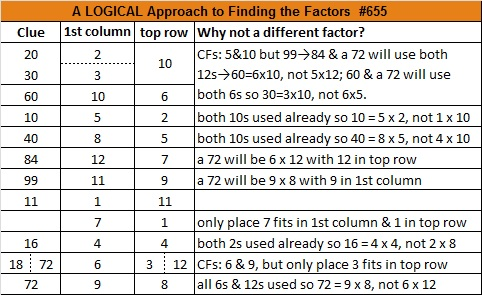# 629 and Level 1

629 is the sum of the 17 prime numbers from 7 to 71. Both of its prime factors, 17 and 37, are included in that list.

17 and 37 are both 10 numbers away from their average, 27. That means that 629 + 10² = 729 or 27².

25² + 2² = 629 and 23² + 10² = 629. Notice that 629 plus or minus 100 is a square number.

Both of 629’s prime factors have a remainder of one when divided by four so 629 is the hypotenuse of four Pythagorean triples, two of which are primitives.

• 100-621-629, a primitive that reminds me of another primitive, 20-21-29
• 204-595-629, three numbers whose greatest common factor is 17
• 296-555-629, three numbers whose greatest common factor is 37
• 429-460-629, a primitive whose shorter leg is exactly 200 less than its hypotenuse.Print the puzzles or type the solution on this excel file: 10 Factors 2015-09-28

—————————————————————————————————

• 629 is a composite number.
• Prime factorization: 629 = 17 x 37
• The exponents in the prime factorization are 1 and 1. Adding one to each and multiplying we get (1 + 1)(1 + 1) = 2 x 2 = 4. Therefore 629 has exactly 4 factors.
• Factors of 629: 1, 17, 37, 629
• Factor pairs: 629 = 1 x 629 or 17 x 37
• 629 has no square factors that allow its square root to be simplified. √629 ≈ 25.079872.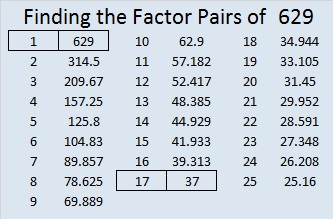—————————————————————————————————# 624 and Level 3

Since 624 is one less than 625 which is 25 squared, it should be obvious that 24 x 26 = 624.

624 is the hypotenuse of the Pythagorean triple 240-576-624. What is the greatest common factor of those three numbers?

624 is the sum of consecutive odd numbers and twin primes 311 and 313.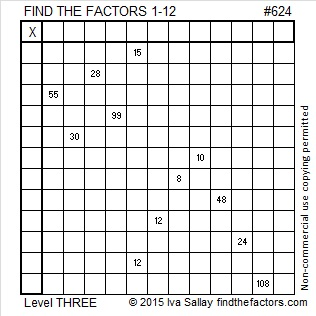Print the puzzles or type the solution on this excel file: 12 Factors 2015-09-21

—————————————————————————————————

• 624 is a composite number.
• Prime factorization: 624 = 2 x 2 x 2 x 2 x 3 x 13, which can be written 624 = (2^4) x 3 x 13
• The exponents in the prime factorization are 4, 1 and 1. Adding one to each and multiplying we get (4 + 1)(1 + 1)(1 + 1) = 5 x 2 x 2 = 20. Therefore 624 has exactly 20 factors.
• Factors of 624: 1, 2, 3, 4, 6, 8, 12, 13, 16, 24, 26, 39, 48, 52, 78, 104, 156, 208, 312, 624
• Factor pairs: 624 = 1 x 624, 2 x 312, 3 x 208, 4 x 156, 6 x 104, 8 x 78, 12 x 52, 13 x 48, 16 x 39 or 24 x 26
• Taking the factor pair with the largest square number factor, we get √624 = (√16)(√39) = 4√39 ≈ 24.97999199.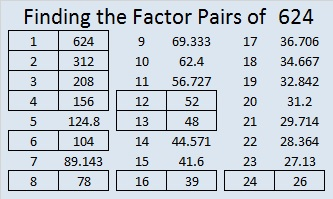—————————————————————————————————

A Logical Approach to solve a FIND THE FACTORS puzzle: Find the column or row with two clues and find their common factor. (None of the factors are greater than 12.)  Write the corresponding factors in the factor column (1st column) and factor row (top row).  Because this is a level three puzzle, you have now written a factor at the top of the factor column. Continue to work from the top of the factor column to the bottom, finding factors and filling in the factor column and the factor row one cell at a time as you go.# 610 and Level 3

The first 17 Fibonacci numbers are 1, 1, 2, 3, 5, 8, 13, 21, 34, 55, 89, 144, 233, 377, 610, 987, 1597. Notice that 610 is the 15th Fibonacci number.

There is a fascinating relationship between some of the Fibonacci numbers and some of the Markov numbers. 610 is the 12th Markov number. Get out your calculator and satisfy yourself that the following two Diophantine equations involving Fibonacci/Markov numbers are true:

1² + 233² + 610² = 3(1)(233)(610)

1² + 610² + 1597² = 3(1)(610)(1597)

Here is a fascinating fact I learned from twitter:

Starting with 5, every other Fibonacci number would be 5, 13, 34, 89, 233, 610, 1597, . . .

610 is on that list. What could be the integer sides of a right triangle with 610 as the hypotenuse?

There are actually FOUR such triangles, namely. . .

• 110-600-610
• 272-546-610
• 414-448-610
• 366-488-610

None of those are primitives, but it is a great list nonetheless!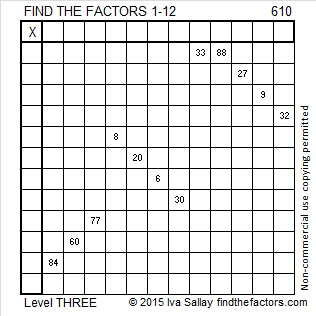Print the puzzles or type the solution on this excel file: 12 Factors 2015-09-07

—————————————————————————————————

• 610 is a composite number.
• Prime factorization: 610 = 2 x 5 x 61
• The exponents in the prime factorization are 1, 1, and 1. Adding one to each and multiplying we get (1 + 1)(1 + 1)(1 + 1) = 2 x 2 x 2 = 8. Therefore 610 has exactly 8 factors.
• Factors of 610: 1, 2, 5, 10, 61, 122, 305, 610
• Factor pairs: 610 = 1 x 610, 2 x 305, 5 x 122, or 10 x 61
• 610 has no square factors that allow its square root to be simplified. √610 ≈ 24.698178.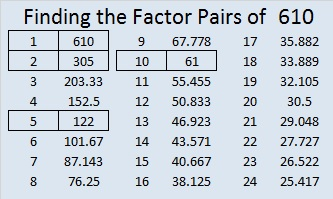—————————————————————————————————

A Logical Approach to solve a FIND THE FACTORS puzzle: Find the column or row with two clues and find their common factor. Write the corresponding factors in the factor column (1st column) and factor row (top row).  Because this is a level three puzzle, you have now written a factor at the top of the factor column. Continue to work from the top of the factor column to the bottom, finding factors and filling in the factor column and the factor row one cell at a time as you go.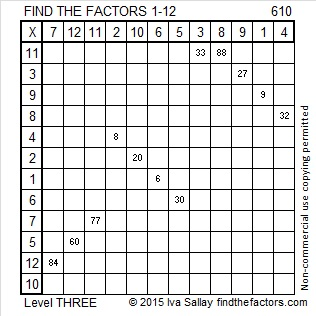# 598 and Level 6

598 is the sum of all the prime number from 17 to 71.

598 is also the hypotenuse of the Pythagorean triple 230-552-598. What is the greatest common factor of those three numbers?

Here’s another easy as 1-2-3 number fact from OEIS.org: (5^1) + (9^2) +(8^3) = 598.

Since 23 + 3 = 26 and 23 x 26 = 598, we know we are only 2 away from 24 x 25 = 600.Print the puzzles or type the solution on this excel file: 12 Factors 2015-08-24

—————————————————————————————————

• 598 is a composite number.
• Prime factorization: 598 = 2 x 13 x 23
• The exponents in the prime factorization are 1, 1, and 1. Adding one to each and multiplying we get (1 + 1)(1 + 1)(1 + 1) = 2 x 2 x 2 = 8. Therefore 598 has exactly 8 factors.
• Factors of 598: 1, 2, 13, 23, 26, 46, 299, 598
• Factor pairs: 598 = 1 x 598, 2 x 299, 13 x 46, or 23 x 26
• 598 has no square factors that allow its square root to be simplified. √598 ≈ 24.4540385.—————————————————————————————————# 596 Select the Correct Top Row

596 is the sum of the four primes numbers from 139 to 157. The two prime numbers in the middle of that sum are twin primes. Do you know what they are?

596 is also the hypotenuse of the Pythagorean triple 204-560-596. What is the greatest common factor of those three numbers?Print the puzzles or type the solution on this excel file: 12 Factors 2015-08-24

—————————————————————————————————

• 596 is a composite number.
• Prime factorization: 596 = 2 x 2 x 149, which can be written 596 = (2^2) x 149
• The exponents in the prime factorization are 1 and 2. Adding one to each and multiplying we get (1 + 1)(2 + 1) = 2 x 3  = 6. Therefore 596 has exactly 6 factors.
• Factors of 596: 1, 2, 4, 149, 298, 596
• Factor pairs: 596 = 1 x 596, 2 x 298, or 4 x 149
• Taking the factor pair with the largest square number factor, we get √596 = (√4)(√149) = 2√149 ≈ 24.41311123—————————————————————————————————# 595 and Level 3

595 = (34/2)(34 + 1) so 595 is the 34th triangular number.

Thus 1 + 2 + 3 + 4 + . . . . . + 31 + 32 + 33 + 34 = 595

595 is also the hypotenuse of four Pythagorean triples. Each of these triples has its own greatest common factor.

• 91-588-595
• 252-539-595
• 280-525-595
• 357-476-595Print the puzzles or type the solution on this excel file: 12 Factors 2015-08-24

—————————————————————————————————

• 595 is a composite number.
• Prime factorization: 595 = 5 x 7 x 17
• The exponents in the prime factorization are 1, 1, and 1. Adding one to each and multiplying we get (1 + 1)(1 + 1)(1 + 1) = 2 x 2 x 2 = 8. Therefore 595 has exactly 8 factors.
• Factors of 595: 1, 5, 7, 17, 35, 85, 119, 595
• Factor pairs: 595 = 1 x 595, 5 x 119, 7 x 85, or 17 x 35
• 595 has no square factors that allow its square root to be simplified. √595 ≈ 24.39262.—————————————————————————————————

A Logical Approach to solve a FIND THE FACTORS puzzle: Find the column or row with two clues and find their common factor. Write the corresponding factors in the factor column (1st column) and factor row (top row).  Because this is a level three puzzle, you have now written a factor at the top of the factor column. Continue to work from the top of the factor column to the bottom, finding factors and filling in the factor column and the factor row one cell at a time as you go.